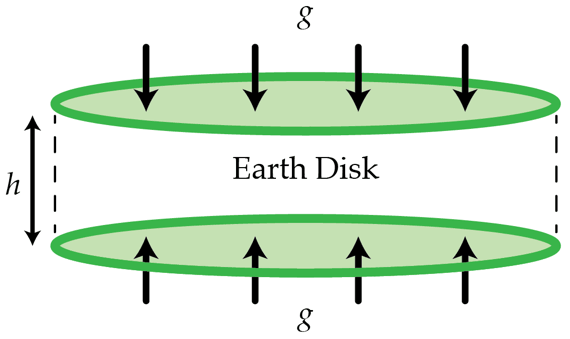# If the Earth were flat...

There was a time, long ago, when people thought that the Earth was flat. Let us suppose that the Earth is a flat disk with density $\rho=5.5\times 10^{3}~\text{kg}/\text{m}^3$. The acceleration due to gravity, right at the surface of the planet, and close to center of the disk is $g=9.8~\text{m}/\text{s}^2$. Determine the thickness $h$ in kilometers of the disk. You may assume that $h\ll R$, where $R$ is the radius of the disk.Details and assumptions

The universal gravitational constant is $G=6.67\times 10^{-11}~\text{m}^{3}\text{kg}^{-1} \text{s}^{-2}$

×

Problem Loading...

Note Loading...

Set Loading...